Next: Regression coefficients Up: Results Previous: Results

## Single-particle energies in function of coupling constants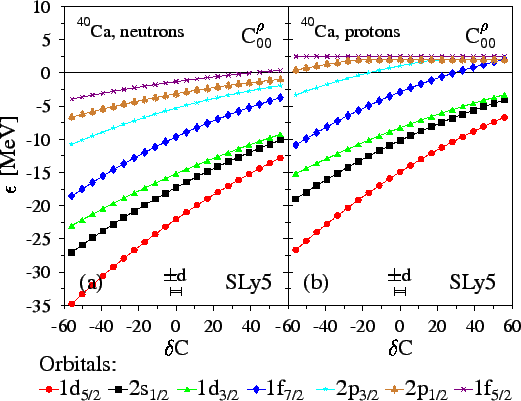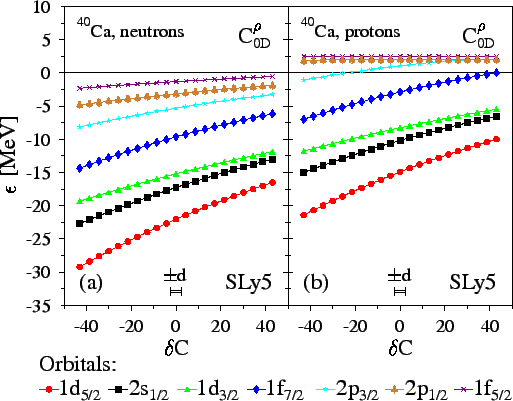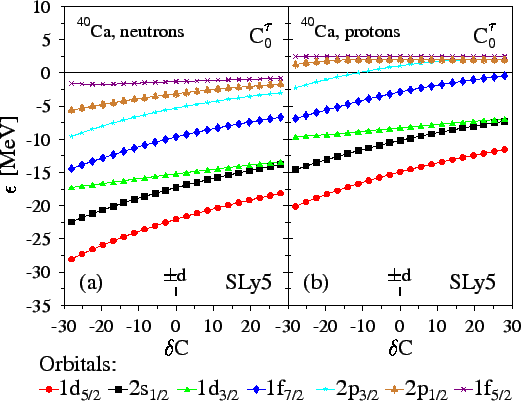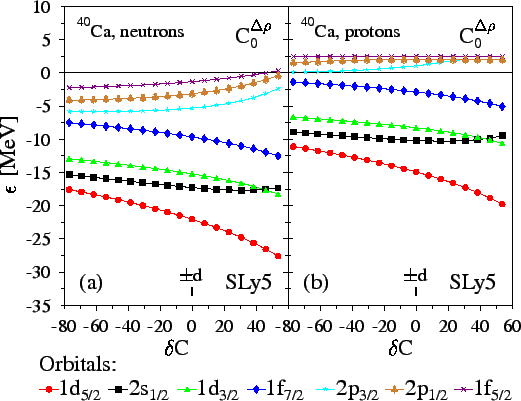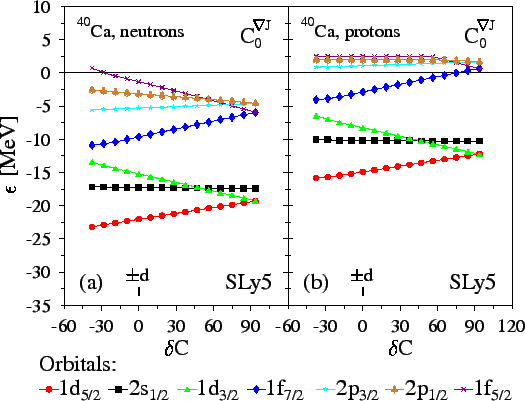In Figs. 1-5 we show the s.p. energies in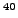Ca as functions of five isoscalar coupling constants (5). Results were obtained by varying one coupling constant of the SLy5 functional and by keeping all the remaining ones at their SLy5 values. Ranges of variation of the coupling constants were chosen in a maximum possible way, i.e., up to the values where changes of level ordering or levels becoming unbound precluded obtaining meaningful solutions. Of course, physical values of these coupling constants are fairly well fixed by adjustments to empirical data, so, in practice, physically acceptable variations must not be so large. Therefore, values of parameters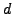used in the regression analysis below, also shown in Figs. 1-5, were quite small. Positive s.p. energies are shown only to indicate in which regions of parameters the levels become unbound - precise values of these s.p. energies are only loosely related to positions of resonances.

In Figs. 1-5 one can clearly see that there is only a rather small overall non-linearity of the s.p. energies as functions of the coupling constants. With decreasing values of coupling constants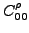and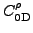, the s.p. potentials become deeper and thus the values of s.p. energies uniformly decrease (Figs. 1 and 2). There are only very small differences in the dependencies induced by varying coupling constantsand. Somewhat larger changes in relative positions of s.p. levels are induced by varying coupling constant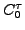(Fig. 3). Decreasing values of this coupling constant induce deeper s.p. potentials and larger values of the effective mass. Coupling constant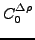clearly influences the surface properties of the s.p. potentials (Fig. 4) by changing relative positions of the low-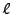and high-levels. Finally, the SO coupling constant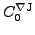very linearly changes the SO splitting of levels shown in Fig. 5. Dependence of levels on the tensor coupling constant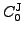is very weak in a spin-saturated nucleusCa, and, therefore, it is not shown.Next: Regression coefficients Up: Results Previous: Results
Jacek Dobaczewski 2008-05-18